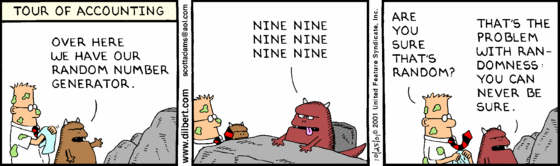# Probability, Statistics and Stochastic Processes, Jul-Nov 2022

### Note

If you want to register for this course, you will need to contact the Academic section. The instructors have no role/say in enrolling students for the class.

### Instructors and Branches

Instructor Branches Room
Dr. Sivaram AE+BE+PH RJN102
Dr. Shaiju EE+MM RJN202
Dr. Vetrivel CE+CH CS15
Dr. Santanu CS+EP+NA CRC305

### Syllabus

Probability Axiomatic definition of probability, Independent Events, Baye's theorem, Discrete and continuous random variables, Distribution, Functions of random variables, Expectation, Variance, Correlation coefficient, Chebyshev’s inequality, Markov inequality, Conditional expectations, Standard discrete and continuous distributions (Binomial, Poisson, Geometric, Exponential, Normal, Chi-square), Moment generating function, Sums of random variables, Law of large numbers, Central limit theorem, Normal approximation to Binomial.

Statistics Sampling distribution, Estimation of parameters, Maximum likelihood estimates, Confidence intervals, Student’s t-distribution, Testing hypothesis, Goodness of fit.

Stochastic Processes Discrete random process, Stationary random process, Bernoulli process, Poisson process, Markov chain.

### Textbooks

• Introduction to Probability, by Dimitri Bertsekas and John N. Tsitsiklis (referred as BT in online lectures)

### Other Reference Textbooks

• Introductory Probability and Statistical Applications, by Paul L. Meyer (referred as PLM in lectures)
• John E. Freund's Mathematical Statistics with Applications, by Irwin Miller and Marylees Miller (referred as M$$^2$$ in lectures)
• Fundamentals of Applied Probability and Random Processes, by Oliver C. Ibe (referred as Ibe in lectures)

### Exams

Date and time of exam, check institute calendar.
Quiz - I Quiz - II Endsem
20 20 60

### Problem Sets

Problem Set 1 Problem Set 1 solution

Problem Set 2 Problem Set 2 solution

Problem Set 3 Problem Set 3 solution

Problem set 4 Problem set 4 solution

Problem set 5 Problem set 5 solution

Problem set 6 Problem set 6 solution part I Problem set 6 solution part II

Problem set 7 Problem set 7 solution

Problem set 8 Problem set 8 solution

### Other "random" stuffGeorge Burns on TV being interviewed on his 100th birthday: George Burns was puffing on a cigar and the interviewer made a comment about the negative correlation between longevity and smoking.
George Burns: "Twenty years ago my doctor told me that these cigars were going to kill me in the next five years with a probability of 99%"
Interviewer: "What does he say now?"
George Burns: "I don't know. He's dead"